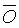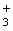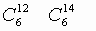CHEMISTRY

 Time: 1 Hour Max.Marks : 60

Instructions:

(1) Answer must be written either in English or the medium of instruction of the candidate in high school.
(2) There will be no negative marking.
(3) Use of calculators or graph papers is not permitted.
(4) There are FOUR Questions. Answer all the questions.

1. The questions below (1-10) consist of an `Assertion’ in column 1 and the `Reason’ in column 2. Use the following key to choose the appropriate answer.

[10x1½=15 Marks]

1. If both assertion and reason are CORRECT, and reason is the CORRECT explanation of the assertion.
2. If both assertion and reason are CORRECT, but reason is NOT the CORRECT explanation of the assertion.
3. If assertion is CORRECT, but reason is INCORRECT.
4. If assertion is INCORRECT, but reason is CORRECT.
 Assertion (column 1) Reason (column 2)
 1 The rate at which sugar dissolves in water increases with stirring Stirring exposes the surface of solute crystal to a less concentrated layer of solution 2 Diamond has a higher melting point In a diamond crystal, the carbon atoms are held in place by ionic bonds 3 Potassium has a lower Ist ionisation energy than lithium has Potassium has more protons in its nucleus than lithium has 4 Zinc metal will reduce Cu2+ in solution Zinc is a more reactive metal than copper 5 CH3COOH+H2O == CH3CO+H3O+ If some acetic acid is added to the equilibrium mixture represented by the equation above, the concentration of HO decreases. The equilibrium constant of a reaction changes as the concentration of reactant changes 6 Fluorine is the highest electronegative element Electro-negativity is the inherent property of the free atom of an element 7 Aromatic compounds give more soot than aliphatic compounds Aromatic compounds are highly inflammable compounds 8have different chemical properties They have the same number of electrons and also similar electronic configuration 9 Heat of neutralisation for both HNO3 and HCl with NaOH is 53.7kJ mole-1 The bond strength in O-H in HNO3 and H-Cl in HCl are equal 10 Bond angle in methane is 1090 28’ Mixing of the atomic orbitals prior to overlap which changes the special distribution of orbitals

II. Explain the following Facts: [10x2=20 Marks]

1. SO2 has dipole moment but CO2 has no dipole moment.

2. Amino acids are covalent compounds but exhibit properties like ionic salts.

3. N3- and O2- are isoelectronic but N3- is larger in size than O2-.

4. Oxygen, Nitrogen and carbon have unpaired electrons 2,3,2 respectively. Their valencies are 2, 3 and 4 respectively.

5. All minerals are not used to extract the metal.

6. Melting point and Boiling point of Ionic compounds are higher than the covalent compound.

7. Glass is called as super cooled liquid.

8. pH of aq.solution of NaCl is 7, but aq.solution of NaF is greater than 7.

9. KOH is much more soluble in liquid NH3 containing water than in pure liquid Ammonia.

10. Iron is mostly used in the form of steel.

III. Differentiate the following: [10x2=20 Marks]

1. drugs - pharmaceuticals

2. magic numbers - quantum numbers

3. molecular formula - structural formula

4. amino acid - proteins

5. heat of reaction - activation energy

6. mixture - compound

7. emulsion - suspensions

8. displacement reaction - double decomposition reaction

9. mono saccharides - polysaccharides

10. barometer - manometer

VI. Solve the following: [5 Marks]

1. A friend is trying to balance the following equation

N2 + H2 ® NH3
He presents you with his version of the balanced equation N + H3
® NH3 . You immediately recognize that he has committed a serious error; however, he argues that there is nothing wrong, since the equation is balanced. What reason can you give to convince him that his method of balancing the equation is flawed. 1M

2. Titanium dioxide is used as the base powder for a variety of cosmetics. Say you decide to manufacture TiO2 in a furnace by the reaction Ti + O2 ® TiO2 . Your titanium supplier can supply titanium at Rs.2.00 per gram. In your process, the reaction has a 33% yield. Market forces demand that you sell your TiO2 product at a cost no more than Rs.3.50 per gram.

1. Assuming that you can sell as much TiO2 as you can make and the O2 is freely available from the atmosphere, is it profitable for you to manufacture TiO2?
2. If it is not profitable to make TiO2, what do you need to charge, per gram, in order to break even, assuming no other costs than the Ti metal?
3. If you can’t increase the price of the TiO2, yet you still want to make a profit, what specific thing do you need to do with your process?(Atomic Wt. Ti=48) 2M

3. Carborundum is silicon carbide, SiC, a very hard material used as an abrasive on sandpaper and in other applications. It is prepared by the reaction of pure sand, SiO2, with carbon at high temperature. Carbon monoxide, CO, is the other product of this reaction. Write the balanced equation for the reaction, and calculate how much SiO2 is required to produce 3.00 kg of SiC. (Atomic Wt. Si = 28) 2M